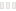Este site usa cookies para fornecer nossos serviços, melhorar o desempenho, para análises e (se não estiver conectado) para publicidade. Ao usar o LibraryThing, você reconhece que leu e entendeu nossos Termos de Serviço e Política de Privacidade . Seu uso do site e dos serviços está sujeito a essas políticas e termos.

# Etiqueta: math fiction

Inclui: math fiction, mathematical fiction, mathfiction, math-fiction, mathematics fiction, mathematical-fiction, Mathematics - Fiction, _fiction (mathematics), Mathematics - fiction, Mathematics--Fiction, Math - Fiction, Maths Fiction, Mathematical Fiction, Mathematics-Fiction, math (fiction), Fiction Math (47 mais), Mathematical fiction, fiction mathematics, math fic, Mathematics-fiction, mathy fiction, Maths fiction, Fiction; Math, math_fiction, MATHEMATICS FICTION, math -fiction, mathematics (fiction), Math fiction, Math/Fiction, Fiction - Mathematics, fiction; Math, mathematics--fiction, Math--Fiction, Mathematics fiction, fiction--mathematics, Math Fiction, Fiction/Math, fiction - math, mathematics-fiction, Fiction (Math), maths fiction, Math-Fiction, Mathematics Fiction, mathematics - fiction, mathematic fiction, fiction-mathematics, math--fiction, Mathematics › Fiction, Math-fiction, Mathematics -Fiction, mathematics -fiction, fiction; math, Math--fiction, fiction (math), fiction math, math - fiction, Fiction - Math, fiction (mathematics), math/fiction, fiction/math, mathematics › fiction, Fiction Mathematics, fiction - mathematicsMostrar detalhes

 seguinte ›
• (27 vezes)
• (12 vezes)
• (8 vezes)
• (7 vezes)
• (7 vezes)
• (7 vezes)
• (7 vezes)
• (7 vezes)
• (6 vezes)
• (6 vezes)
• (6 vezes)
• (5 vezes)
• (5 vezes)
• (5 vezes)
• (5 vezes)
• (5 vezes)
• (4 vezes)
• (4 vezes)
• (4 vezes)
• (4 vezes)
• (4 vezes)
• (4 vezes)
• (4 vezes)
• (4 vezes)
• (4 vezes)

## Usando a etiqueta

fantasiakirja (65), kirja (62), magid (22), jeanined (14), qebo (12), souci (11), sherryearly (10), Friendshaverford (10), kirjatest (8), austin.dixon (8), alexenglishauthor (6), OldMillCenter (6), muumi (5), meat (4), lorieshiveley (4), tortoise (4), albusseverus (3), EowynA (3), MerrittGibsonLibrary (3), DavidFosterWallace (3), alu042 (2) ... e 388 outros membros